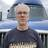VLOOKUP formula between 2 tables

Hello,

I'm looking for a vlookup formula to get information stored in another table.

- Table 1 with EAN Code (for example 5400101123456) and Product name (for example spaghetti).

- Table 2 with EAN Code (same as Table 1 - 5400101123456) and a formula field.

Which formula can i put in the formula field (into table 2) to get "spaghetti" (from table 1) as result?

David W.This works...

`(select Table1 where 'EAN Code' = 'EAN Code').'Product Name'`

Can you please clarify for the 'EAN Code': from Table 1 or from Table 2

Also, if from a different table, how can i right the correct syntax?

In my case:

Table 1 is Fournisseur

Table 2 is SensoPro

EAN code table 1 is IDSAP

EAN code table 2 is IDFOURN

The result needed is IDGESTPRO in table 1

Thanks for your help and have a nice day,

David W.Hi David, The following should work and, according to your description, would go into the Formula field in SensoPro...

`(select Fournisseur where IDSAP = IDFOURN).IDGESTPRO`

if not, try this...

`let myEAN = this.IDFOURN;`

`(select Fournisseur where IDSAP = myEAN).IDGESTPRO`

Thanks Sean, the second formula works.

I only had to write let myEAN := this.IDFOURN...

David W.